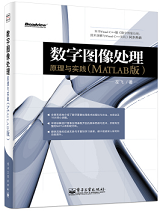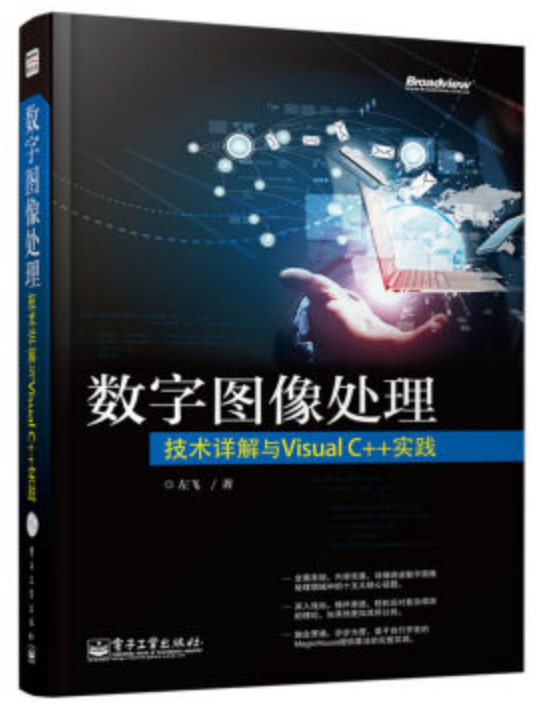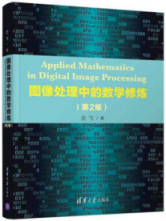# 图像处理tv方法

## 图像处理与机器视觉初学者学习路线

2018-09-06 14:09:07 weixin_42717711 阅读数 13016
• ###### 色彩空间转换

通过本课程的学习，可以对数字成像系统知识整体上得到理解，在相机等成像产品的开发中，获得理论与实际的帮助。

743人学习 姜卓
免费试看

1、毕业于荷兰特温特大学的Dirk-Jan Kroon博士，在Mathworks的FileExchange上的链接，曾经到访过他原来读书时的主页，当时有句话对他的评价是，他非常喜欢计算机编程，这个真不假，下面这个链接里有他用Matlab写成的近百个程序源码，质量非常高，而且涉猎广泛。

http://www.mathworks.com/matlabcentral/fileexchange/authors/29180

2、这是研究 image matting（中文叫抠图——这名字真难听，不知道是谁始作俑者）必去的一个网站，里面有大量关于这个主题内容的介绍，包括最新的成果，评测和对比。
http://www.alphamatting.com/

3、laviewpbt的专栏，他有两个基本同步的博客，一个在CSDN，一个在博客园，自称是“一心无二用，本人只专注于基础图像算法的实现与优化，如图像增强、滤镜、分割、解码编码等，无心恋及图像识别。 ”。博客中很少有提供完整的源代码，但是对理论算法的介绍非常到位，我也同意博主的看法，如果真的对算法理解到位了，写代码处理并不是难事。
http://www.cnblogs.com/Imageshop/

4、Rachel Zhang的专栏（浙大计算机女硕士），CSDN博客排名百名以内的名博，里面有大量图像处理和计算机视觉的资料，有算法讲解，也有很多代码实现（OpenCV居多，少量Matlab）
http://blog.csdn.net/abcjennifer/article/category/1173803/2

5、小魏的修行路（又一个女学霸，博主应该是北大女硕士），博客都是图文并茂的，很详细很用心，代码实现上也是用OpenCV的居多。
http://blog.csdn.net/xiaowei_cqu/

6、非常棒的网站，超多资源。IPOL is a research journal of image processing and image analysis. Each article contains a text on an algorithm and its source code。讨论了超过20个大的Topics，具体每个Topics里面还有许多具体的实现分支，配有可供研究的源代码。研究图像处理不可不看的网站。

http://www.ipol.im/

http://www.librow.com/articles

8、采石工的博客

http://www.cnblogs.com/quarryman/

1、KAZE特征检测作者的主页，里面有文章，也有代码实现。Pablo F. Alcantarilla博士人非常Nice，我给他写Email讨论问题，基本上8个小时之内就能收到回复，也非常感谢他提供的一些参考资料。

http://www.robesafe.com/personal/pablo.alcantarilla/kaze.html

2、在去噪领域中当前最成功的算法莫过于BM3D系列（当然还有BM4D等等），下面这个是项目的主页，非常值得推荐，对于研究降噪问题的同学实在应该仔细看看。

http://www.cs.tut.fi/~foi/GCF-BM3D/

3、布朗大学Douglas Lanman博士的主页，很多有意思的成果，部分有代码资源下载
http://mesh.brown.edu/dlanman/courses.html

4、两位以色列学者（犹太人）的主页。经验中，大部分Paper的作者会在自己的网站上贴出文章，但很少附有代码，如果你自己去写个代码，很多都无法达到作者paper中给出的效果，吹水的可能性极大。但是在研究Close-form soluting 的图像matting时，看到了以色列女学者Anat Levin的主页，作者就提供有matlab代码，很值得推荐。
http://www.wisdom.weizmann.ac.il/~levina/

http://visl.technion.ac.il/~gilboa/PDE-filt/tv_denoising.html

## 【图像处理】全分发TV图像去噪

2014-01-20 14:57:46 xiaowei_cqu 阅读数 13523
• ###### 色彩空间转换

通过本课程的学习，可以对数字成像系统知识整体上得到理解，在相机等成像产品的开发中，获得理论与实际的帮助。

743人学习 姜卓
免费试看

```function J=tv(I,iter,dt,ep,lam,I0,C)
%% Private function: tv (by Guy Gilboa).
%% Total Variation denoising.
%% Example: J=tv(I,iter,dt,ep,lam,I0)
%% Input: I    - image (double array gray level 1-256),
%%        iter - num of iterations,
%%        dt   - time step [0.2],
%%        ep   - epsilon (of gradient regularization) ,
%%        lam  - fidelity term lambda ,
%%        I0   - input (noisy) image [I0=I]
%%       (default values are in [])
%% Output: evolved image

if ~exist('ep')
ep=1;
end
if ~exist('dt')
dt=ep/5;  % dt below the CFL bound
end
if ~exist('lam')
lam=0;
end
if ~exist('I0')
I0=I;
end
if ~exist('C')
C=0;
end
[ny,nx]=size(I); ep2=ep^2;

for i=1:iter,  %% do iterations
% estimate derivatives
I_x = (I(:,[2:nx nx])-I(:,[1 1:nx-1]))/2;
I_y = (I([2:ny ny],:)-I([1 1:ny-1],:))/2;
I_xx = I(:,[2:nx nx])+I(:,[1 1:nx-1])-2*I;
I_yy = I([2:ny ny],:)+I([1 1:ny-1],:)-2*I;
Dp = I([2:ny ny],[2:nx nx])+I([1 1:ny-1],[1 1:nx-1]);
Dm = I([1 1:ny-1],[2:nx nx])+I([2:ny ny],[1 1:nx-1]);
I_xy = (Dp-Dm)/4;
% compute flow
Num = I_xx.*(ep2+I_y.^2)-2*I_x.*I_y.*I_xy+I_yy.*(ep2+I_x.^2);
Den = (ep2+I_x.^2+I_y.^2).^(3/2);
I_t = Num./Den + lam.*(I0-I+C);
I=I+dt*I_t;  %% evolve image by dt
end % for i
%% return image
%J=I*Imean/mean(mean(I)); % normalize to original mean
J=I;

```

```  //TV去噪函数
Mat TVDenoising(Mat img, int iter)
{
int ep = 1;
int nx=img.cols;
int ny = img.rows;
double dt = 0.25f;
double lam = 0.0;
int ep2 = ep*ep;

double** image = newDoubleMatrix(nx, ny);
double** image0 = newDoubleMatrix(nx, ny);

for(int i=0;i<ny;i++){
uchar* p=img.ptr<uchar>(i);
for(int j=0;j<nx;j++){
image0[i][j]=image[i][j]=(double)p[j];
}
}
//double** image_x = newDoubleMatrix(nx, ny);   //I_x = ( I(:,[2:nx nx]) - I(:,[1 1:nx-1]))/2;
//double** image_xx = newDoubleMatrix(nx, ny);   //I_xx = I(:,[2:nx nx])+I(:,[1 1:nx-1])-2*I;
//double** image_y = newDoubleMatrix(nx, ny);   //I_y = (I([2:ny ny],:)-I([1 1:ny-1],:))/2;
//double** image_yy = newDoubleMatrix(nx, ny);   //I_yy = I([2:ny ny],:)+I([1 1:ny-1],:)-2*I;
//double** image_dp = newDoubleMatrix(nx, ny);   //Dp = I([2:ny ny],[2:nx nx])+I([1 1:ny-1],[1 1:nx-1
//double** image_dm = newDoubleMatrix(nx, ny);   //Dm = I([1 1:ny-1],[2:nx nx])+I([2:ny ny],[1 1:nx-1]);
//double** image_xy = newDoubleMatrix(nx, ny);   //I_xy = (Dp-Dm)/4;
//double** image_num = newDoubleMatrix(nx, ny);   //Num = I_xx.*(ep2+I_y.^2)-2*I_x.*I_y.*I_xy+I_yy.*(ep2+I_x.^2);
//double** image_den = newDoubleMatrix(nx, ny);   //Den = (ep2+I_x.^2+I_y.^2).^(3/2);

//////////////////////////////////////////////////////////////////////////
//对image进行迭代iter次
//iter = 80;
for (int t = 1; t <= iter; t++){

//for (int i = 0; i < ny; i++){
//	for (int j = 0; j < nx; j++){
//		//I_x  = (I(:,[2:nx nx])-I(:,[1 1:nx-1]))/2;
//		//I_y  = (I([2:ny ny],:)-I([1 1:ny-1],:))/2;
//		//I_xx = I(:,[2:nx nx])+I(:,[1 1:nx-1])-2*I;
//		//I_yy = I([2:ny ny],:)+I([1 1:ny-1],:)-2*I;
//		//Dp   = I([2:ny ny],[2:nx nx])+I([1 1:ny-1],[1 1:nx-1]);
//		//Dm   = I([1 1:ny-1],[2:nx nx])+I([2:ny ny],[1 1:nx-1]);
//		//I_xy = (Dp-Dm)/4;
//		int tmp_i1=(i+1)<ny ? (i+1) :(ny-1);
//		int tmp_j1=(j+1)<nx ? (j+1): (nx-1);
//		int tmp_i2=(i-1) > -1 ? (i-1) : 0;
//		int tmp_j2=(j-1) > -1 ? (j-1) : 0;
//		image_x[i][j] = (image[i][tmp_j1] - image[i][tmp_j2])/2;
//		image_y[i][j]= (image[tmp_i1][j]-image[tmp_i2][j])/2;
//		image_xx[i][j] = image[i][tmp_j1] + image[i][tmp_j2]- image[i][j]*2;
//		image_yy[i][j]= image[tmp_i1][j]+image[tmp_i2][j] - image[i][j]*2;
//		image_dp[i][j]=image[tmp_i1][tmp_j1]+image[tmp_i2][tmp_j2];
//		image_dm[i][j]=image[tmp_i2][tmp_j1]+image[tmp_i1][tmp_j2];
//		image_xy[i][j] = (image_dp[i][j] - image_dm[i][j])/4;
//		image_num[i][j] = image_xx[i][j]*(image_y[i][j]*image_y[i][j] + ep2)
//			- 2*image_x[i][j]*image_y[i][j]*image_xy[i][j] + image_yy[i][j]*(image_x[i][j]*image_x[i][j] + ep2);
//		image_den[i][j] = pow((image_x[i][j]*image_x[i][j] + image_y[i][j]*image_y[i][j] + ep2), 1.5);
//		image[i][j] += dt*(image_num[i][j]/image_den[i][j] + lam*(image0[i][j] - image[i][j]));
//	}
//}
for (int i = 0; i < ny; i++){
for (int j = 0; j < nx; j++){
int tmp_i1=(i+1)<ny ? (i+1) :(ny-1);
int tmp_j1=(j+1)<nx ? (j+1): (nx-1);
int tmp_i2=(i-1) > -1 ? (i-1) : 0;
int tmp_j2=(j-1) > -1 ? (j-1) : 0;
double tmp_x = (image[i][tmp_j1] - image[i][tmp_j2])/2; //I_x  = (I(:,[2:nx nx])-I(:,[1 1:nx-1]))/2;
double tmp_y= (image[tmp_i1][j]-image[tmp_i2][j])/2; //I_y  = (I([2:ny ny],:)-I([1 1:ny-1],:))/2;
double tmp_xx = image[i][tmp_j1] + image[i][tmp_j2]- image[i][j]*2; //I_xx = I(:,[2:nx nx])+I(:,[1 1:nx-1])-2*I;
double tmp_yy= image[tmp_i1][j]+image[tmp_i2][j] - image[i][j]*2; //I_yy = I([2:ny ny],:)+I([1 1:ny-1],:)-2*I;
double tmp_dp=image[tmp_i1][tmp_j1]+image[tmp_i2][tmp_j2]; //Dp   = I([2:ny ny],[2:nx nx])+I([1 1:ny-1],[1 1:nx-1]);
double tmp_dm=image[tmp_i2][tmp_j1]+image[tmp_i1][tmp_j2]; //Dm   = I([1 1:ny-1],[2:nx nx])+I([2:ny ny],[1 1:nx-1]);
double tmp_xy = (tmp_dp - tmp_dm)/4; //I_xy = (Dp-Dm)/4;
double tmp_num = tmp_xx*(tmp_y*tmp_y + ep2)
- 2*tmp_x*tmp_y*tmp_xy +tmp_yy*(tmp_x*tmp_x + ep2); //Num = I_xx.*(ep2+I_y.^2)-2*I_x.*I_y.*I_xy+I_yy.*(ep2+I_x.^2);
double tmp_den= pow((tmp_x*tmp_x + tmp_y*tmp_y + ep2), 1.5); //Den = (ep2+I_x.^2+I_y.^2).^(3/2);
image[i][j] += dt*(tmp_num/tmp_den+ lam*(image0[i][j] - image[i][j]));
}
}

}

Mat new_img;
img.copyTo(new_img);
for(int i=0;i<img.rows;i++){
uchar* p=img.ptr<uchar>(i);
uchar* np=new_img.ptr<uchar>(i);
for(int j=0;j<img.cols;j++){
int tmp=(int)image[i][j];
tmp=max(0,min(tmp,255));
np[j]=(uchar)(tmp);
}
}

//////////////////////////////////////////////////////////////////////////
//删除内存
//deleteDoubleMatrix(image_x, nx, ny);
//deleteDoubleMatrix(image_y, nx, ny);
//deleteDoubleMatrix(image_xx, nx, ny);
//deleteDoubleMatrix(image_yy, nx, ny);
//deleteDoubleMatrix(image_dp, nx, ny);
//deleteDoubleMatrix(image_dm, nx, ny);
//deleteDoubleMatrix(image_xy, nx, ny);
//deleteDoubleMatrix(image_num, nx, ny);
//deleteDoubleMatrix(image_den, nx, ny);
deleteDoubleMatrix(image0, nx, ny);
deleteDoubleMatrix(image, nx, ny);

//imshow("Image",img);
//imshow("Denosing",new_img);

return new_img;
}```

## 【图像修复】浅析：图像修复中的TV模型

2015-03-14 10:12:22 hujingshuang 阅读数 13292
• ###### 色彩空间转换

通过本课程的学习，可以对数字成像系统知识整体上得到理解，在相机等成像产品的开发中，获得理论与实际的帮助。

743人学习 姜卓
免费试看①matlab源码实现：

```img=double(imread('lena.jpg'));
[m n]=size(img);
for i=1:m
for j=1:n
img(i,j)=0;
end
end
end
imshow(img,[]);     %合成的需要修复的图像

lambda=0.2;
a=0.5;%避免分母为0
imgn=img;
for l=1:1500         %迭代次数
for i=2:m-1
for j=2:n-1
Un=sqrt((img(i,j)-img(i-1,j))^2+((img(i-1,j-1)-img(i-1,j+1))/2)^2);
Ue=sqrt((img(i,j)-img(i,j+1))^2+((img(i-1,j+1)-img(i+1,j+1))/2)^2);
Uw=sqrt((img(i,j)-img(i,j-1))^2+((img(i-1,j-1)-img(i+1,j-1))/2)^2);
Us=sqrt((img(i,j)-img(i+1,j))^2+((img(i+1,j-1)-img(i+1,j+1))/2)^2);

Wn=1/sqrt(Un^2+a^2);
We=1/sqrt(Ue^2+a^2);
Ww=1/sqrt(Uw^2+a^2);
Ws=1/sqrt(Us^2+a^2);

Hon=Wn/((Wn+We+Ww+Ws)+lambda);
Hoe=We/((Wn+We+Ww+Ws)+lambda);
How=Ww/((Wn+We+Ww+Ws)+lambda);
Hos=Ws/((Wn+We+Ww+Ws)+lambda);

Hoo=lambda/((Wn+We+Ww+Ws)+lambda);
value = Hon*img(i-1,j)+Hoe*img(i,j+1)+How*img(i,j-1)+Hos*img(i+1,j)+Hoo*img(i,j);
imgn(i,j)= value;
end
end
end
img=imgn;
end
figure;
imshow(img)```
opencv源码实现：

```#include <iostream>
#include <stdlib.h>
#include <cv.h>
#include <math.h>
#include <opencv2/highgui/highgui.hpp>
#include <opencv2/core/core.hpp>
#include <opencv2/imgproc/imgproc.hpp>

using namespace cv;

int main(void)
{
//读取原始图像及掩模图像
//合成需要修复的图像
int i, j;
CvMat *src = cvCreateMat(M, N, CV_32FC1);//存放浮点图像
cvConvert(src_uint8, src);
for (i = 0; i < M; i++)
{
for (j = 0; j < N; j++)
{
{
((float*)(void*)(src->data.ptr + (size_t)src->step*i))[j] = 0.0;
}
if (((float*)(void*)(src->data.ptr + (size_t)src->step*i))[j] < 0)
{
((float*)(void*)(src->data.ptr + (size_t)src->step*i))[j] += 256.0;
}
}
}
cvConvert(src, src_uint8);
cvShowImage("需要修复的图像", src_uint8);
cvWaitKey(0);

double t = getTickCount();//当前滴答数
float lambda = 0.2;
float delta = 0.5;
float UO, UN, UW, US, UE, UNE, UNW, USW, USE;
float Un, Ue, Uw, Us;
float Wn, We, Ww, Ws;
float Hon, Hoe, How, Hos;
float Hoo;
int iteration = 500;
while(iteration)
{
for (i = 1; i < M - 1; i++)
{
for (j = 1; j < N - 1; j++)
{
{
UO = ((float*)(void*)(src->data.ptr + (size_t)src->step*i))[j];
UN = ((float*)(void*)(src->data.ptr + (size_t)src->step*(i-1)))[j];
US = ((float*)(void*)(src->data.ptr + (size_t)src->step*(i+1)))[j];
UE = ((float*)(void*)(src->data.ptr + (size_t)src->step*i))[j+1];
UW = ((float*)(void*)(src->data.ptr + (size_t)src->step*i))[j-1];

UNE = ((float*)(void*)(src->data.ptr + (size_t)src->step*(i-1)))[j+1];
UNW = ((float*)(void*)(src->data.ptr + (size_t)src->step*(i-1)))[j-1];
USE = ((float*)(void*)(src->data.ptr + (size_t)src->step*(i+1)))[j+1];
USW = ((float*)(void*)(src->data.ptr + (size_t)src->step*(i+1)))[j-1];

Un = sqrt((UO - UN) * (UO - UN) + ((UNW - UNE) / 2.0) * ((UNW - UNE) / 2.0));
Ue = sqrt((UO - UE) * (UO - UE) + ((UNE - USE) / 2.0) * ((UNE - USE) / 2.0));
Uw = sqrt((UO - UW) * (UO - UW) + ((UNW - USW) / 2.0) * ((UNW - USW) / 2.0));
Us = sqrt((UO - US) * (UO - US) + ((USW - USE) / 2.0) * ((USW - USE) / 2.0));

Wn = 1.0/sqrt(Un * Un + delta * delta);
We = 1.0/sqrt(Ue * Ue + delta * delta);
Ww = 1.0/sqrt(Uw * Uw + delta * delta);
Ws = 1.0/sqrt(Us * Us + delta * delta);

Hon = Wn/(Wn+We+Ww+Ws+lambda);
Hoe = We/(Wn+We+Ww+Ws+lambda);
How = Ww/(Wn+We+Ww+Ws+lambda);
Hos = Ws/(Wn+We+Ww+Ws+lambda);

Hoo = lambda/(Wn+We+Ww+Ws+lambda);
((float*)(void*)(src->data.ptr + (size_t)src->step*i))[j]=(Hon*UN+Hoe*UE+How*UW+Hos*US+Hoo*UO);
}
}
}
iteration--;
}
cvConvert(src, src_uint8);
t = ((double)getTickCount() - t)/getTickFrequency();
printf("算法用时：%f秒\n", t);
cvShowImage("修复结果", src_uint8);
cvWaitKey(0);
}
```1、本博客例程仅做学习交流用，切勿用于商业用途。

2、欢迎交流，转载请注明出处：http://blog.csdn.net/hujingshuang/article/details/44257179

## 如何学好图像处理——从小白到大神？

2016-02-26 17:48:13 baimafujinji 阅读数 69723
• ###### 色彩空间转换

通过本课程的学习，可以对数字成像系统知识整体上得到理解，在相机等成像产品的开发中，获得理论与实际的帮助。

743人学习 姜卓
免费试看

1950年，美国的麻省理工学院制造出了第一台配有图形显示器的电子计算机——旋风I号（Whirlwind I）。旋风I号的显示器使用一个类似于示波器的阴极射线管（Cathode Ray Tube，CRT）来显示一些简单的图形。1958年美国Calcomp公司研制出了滚筒式绘图仪，GerBer公司把数控机床发展成为平板式绘图仪。在这一时期，电子计算机都主要应用于科学计算，而为这些计算机配置的图形设备也仅仅是作为一种简单的输出设备。

1964年，美国加利福尼亚的喷气推进实验室用计算机对“旅行者七号”太空船发回的大批月球照片进行处理，以校正航天器上摄影机中各种类型的图像畸变，收到了明显的效果。在后来的宇航空间技术中，数字图像处理技术都发挥了巨大的作用。

• 1）图像获取和输出
• 2）图像编码和压缩
• 3）图像增强与复原
• 4）图像的频域变换
• 5）图像的信息安全
• 6）图像的区域分割
• 7）图像目标的识别
• 8）图像的几何变换

• 1）一些专业图像处理软件：Photoshop、CorelDRAW……
• 2）一些手机APP应用：美图秀秀、玩图……
• 3）一些医学图像处理应用：MRI、彩超图像处理……
• 4）一些制造业上的应用：元器件检测、瑕疵检测……
• 5）一些摄像头、相机上的应用：夜间照片的质量改善……
• 6）一些电影工业上是应用：换背景、电影特技……

1）如果你是我上述那些应用领域的从业者，你当然需要掌握图像方面的理论和技术；2）相关专业的研究人员、大专院校的博士生、研究生。

1）对于初级入门者

1）这是一本专门为Electronic Engineering专业学生所写的书。它需要有信号与系统、数字信号处理这两门课作为基础。如果你没有这两门课的基础，你读这本书要么是看热闹，要么就是看不懂。2）冈萨雷斯教授的《数字图像处理》第一版是在1977年出版的，到现在已经快40年了；现在国内广泛使用的第二版是2002年出版的（第三版是2007年但是其实二者差异并不大），到现在也有20年左右的时间了。事实上，冈萨雷斯教授退休也有快30年了。所以这本书的内容已经偏于陈旧。数字图像处理这个领域的发展绝对是日新月异，突飞猛进的。特别在最近二三十年里，很多新思路，新方法不断涌现。如果你看了我前面推荐的Rich教授的公开课（这也是当前美国大学正在教学的内容），你一下子就会发现，原来我们的教育还停留在改革开放之前外国的水平上。这其实特别可怕。所以我觉得冈萨雷斯教授的《数字图像处理》作为学习过程中的一个补充还是不错的，但是如果把它作为主参考，那真的就是：国外都洋枪洋炮了，我们还在大刀长矛。2）对于中级水平者

http://blog.csdn.net/baimafujinji/article/details/32332079

3）对于高级进阶者

（全文完）## TV图像处理详解

2017-04-25 10:16:31 neo_qiye 阅读数 1508
• ###### 色彩空间转换

通过本课程的学习，可以对数字成像系统知识整体上得到理解，在相机等成像产品的开发中，获得理论与实际的帮助。

743人学习 姜卓
免费试看